# Exponentials and Logarithms in Math: Tutoring Solution Chapter Exam

Exam Instructions:

Choose your answers to the questions and click 'Next' to see the next set of questions. You can skip questions if you would like and come back to them later with the yellow "Go To First Skipped Question" button. When you have completed the practice exam, a green submit button will appear. Click it to see your results. Good luck!

### Page 1

#### Question 1 1. Evaluate the following.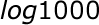#### Question 2 2. Solve, to 2 decimal places, the exponential equation below for x: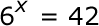#### Question 3 3. Expand the following logarithm: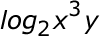### Page 2

#### Question 8 8. Evaluate the following.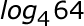#### Question 9 9. Solve the following equation for x.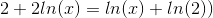#### Question 10 10. Solve the exponential equation below for x: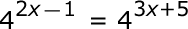### Page 3

#### Question 13 13. Condense the logarithm below: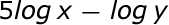#### Question 14 14. Solve the exponential equation below for x: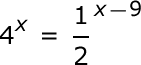### Page 4

#### Question 16 16. Solve the exponential equation below for x: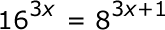#### Question 17 17. Evaluate the following.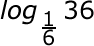#### Question 19 19. Which logarithmic property is shown below?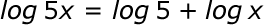### Page 5

#### Question 22 22. Use the following information to answer the question.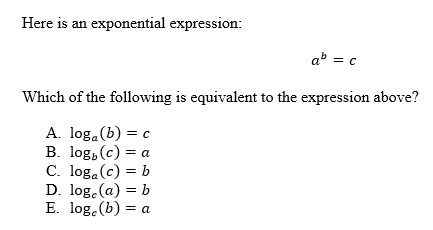#### Question 23 23. Evaluate the logarithm below to 2 decimal places.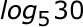#### Question 24 24. Evaluate the logarithm below: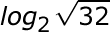#### Question 25 25. Evaluate the logarithm below: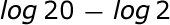### Page 6

#### Exponentials and Logarithms in Math: Tutoring Solution Chapter Exam Instructions

Choose your answers to the questions and click 'Next' to see the next set of questions. You can skip questions if you would like and come back to them later with the yellow "Go To First Skipped Question" button. When you have completed the practice exam, a green submit button will appear. Click it to see your results. Good luck!

Support